Practice
Resources
Contests
Online IDE
New
Free Mock
Scaler
Practice
Improve your coding skills with our resources
Contests
Compete in popular contests with top coders
Scaler
Explore Offerings by SCALER## Welcome to Interviewbit, help us create the best experience for you!

Currently, You are a:College/University *
Enter the name of your college
Branch *
Year of completion *College/University *
Enter the name of your college
Branch *
Year of completion *Current Company *
Enter company name
Experience *## You're all set!Full name *
Email *

By creating an account, I acknowledge that I have read and agree to InterviewBit’s Terms and Privacy Policy .## Welcome back!

Email *Go to Problems

Be a Code Ninja!

# Hashing Techniques

Collisions are bound to occur no matter how good a hash function is. Hence, to maintain the performance of a hash table, it is important to minimise collisions through various collision resolution techniques.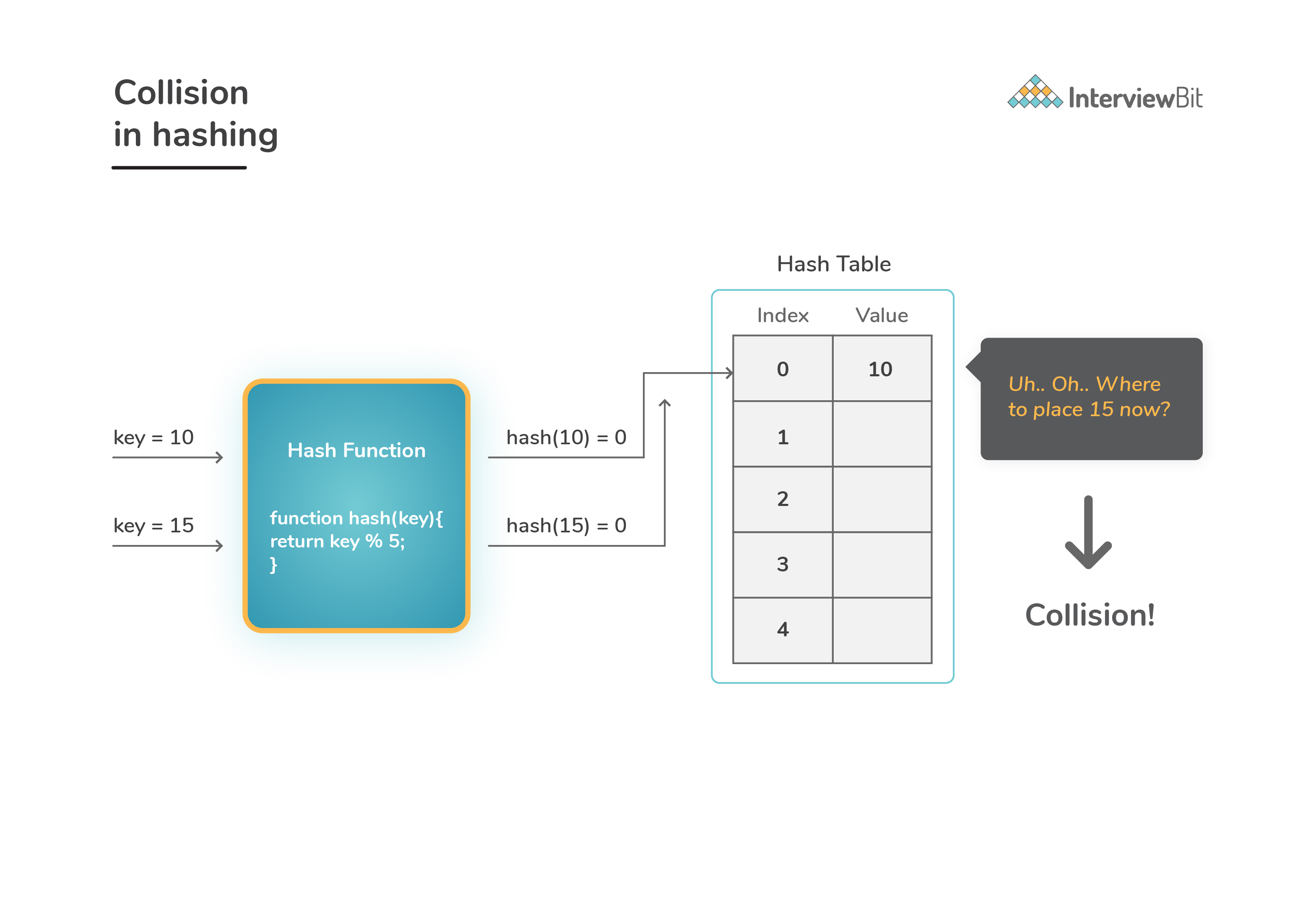There are majorly 2 methods for handling collisions:

• Separate Chaining

### Seperate Chaining

• Here the main idea is to make each slot of hash table point to a linked list of records that have the same hash value.• Performance Analysis:
• Hashing performance can be evaluated under the assumption that each key is equally likely and uniformly hashed to any slot of hash table.
• Consider `table_size` as number of slots in hash table and `n` as number of keys to be saved in the table. Then:
``````We have Load factor α = n/table_size
Time complexity to search/delete = O(1 + α)
Time complexity for insert = O(1)

Hence, overall time complexity of search, insert and delete operation will be O(1) if α is O(1)
``````
• This technique is very simple in terms of implementation.
• We can guarantee that the insert operation always occurs in `O(1)` time complexity as linked lists allows insertion in constant time.
• We need not worry about hash table getting filled up. We can always add any number elements to the chain whenever needed.
• This method is less sensitive or not very much dependent on the hash function or the load factors.
• Generally this method is used when we do not know exactly how many and how frequently the keys would be inserted or deleted.
• Chaining uses extra memory space for creating and maintaining links.
• It might happen that some parts of hash table will never be used. This technically contributes to wastage of space.
• In the worst case scenario, it might happen that all the keys to be inserted belong to a single bucket. This would result in a linked list structure and the search time would be `O(n)`.
• Chaining cache performance is not that great since we are storing keys in the form of linked list. Open addressing techniques has better cache performance as all the keys are guaranteed to be stored in the same table. We will explore open addressing techniques in the next section.

• In this technique, we ensure that all records are stored in the hash table itself. The size of the table must be greater than or equal to the total number of keys available. In case the array gets filled up, we increase the size of table by copying old data whenever needed. How do we handle the following operations in this techniques? Let’s see below:
• Insert(key): When we try to insert a key to the bucket which is already occupied, we keep probing the hash table until an empty slot is found. Once we find the empty slot, we insert `key` into that slot.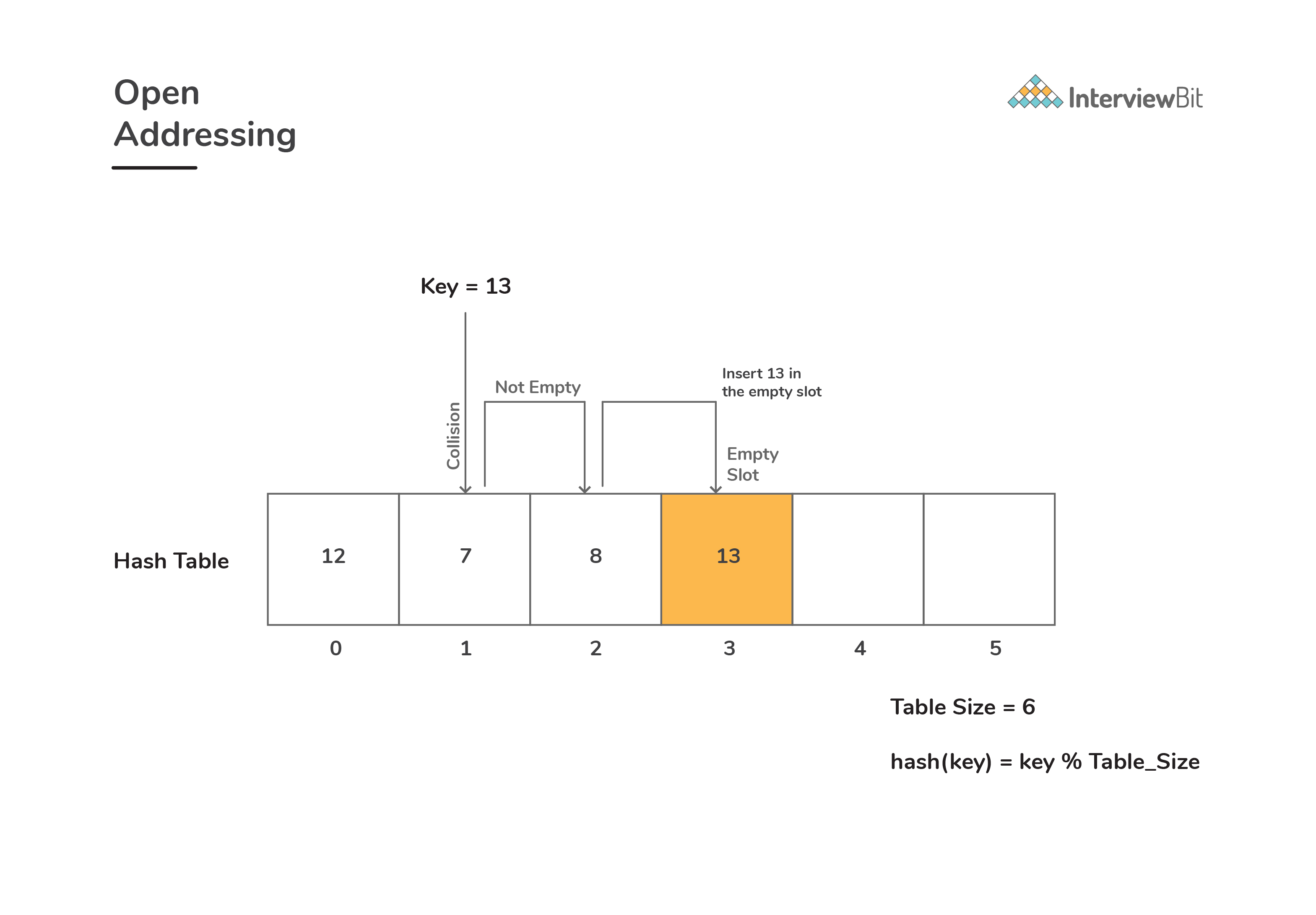• Search(key): While searching for `key` in the hash table, we keep probing until slot’s value doesn’t become equal to `key` or until an empty slot is found.
• Delete(key): While performing delete operation, when we try to simply delete `key`, then the search operation for that key might fail. Hence, deleted key’s slots are marked as “deleted” so that we get the status of the key when searched.
• The term “open addressing” tells us that the address or location of the key to be placed is not determined by its hash value.
• Following are the techniques for following open addressing:
• Linear Probing:

• In this, we linearly probe for the next free slot in the hash table. Generally, gap between two probes is taken as 1.
• Consider `hash(key)` be the slot index computed using hash function and `table_size` represent the hash table size. Suppose `hash(key)` index has a value occupied already, then:
`````` We check if (hash(key) + 1) % table_size is free
If ((hash(key) + 1) % table_size) is also not free, then we check for ((hash(key) + 2) % table_size)
If ((hash(key) + 2) % table_size) is again not free, then we try ((hash(key) + 3) % table_size),
:
:
:
and so on until we find the next available free slot
``````
• When performing search operation, the array is scanned linearly in the same sequence until we find the target element or an empty slot is found. Empty slot indicates that there is no such key present in the table.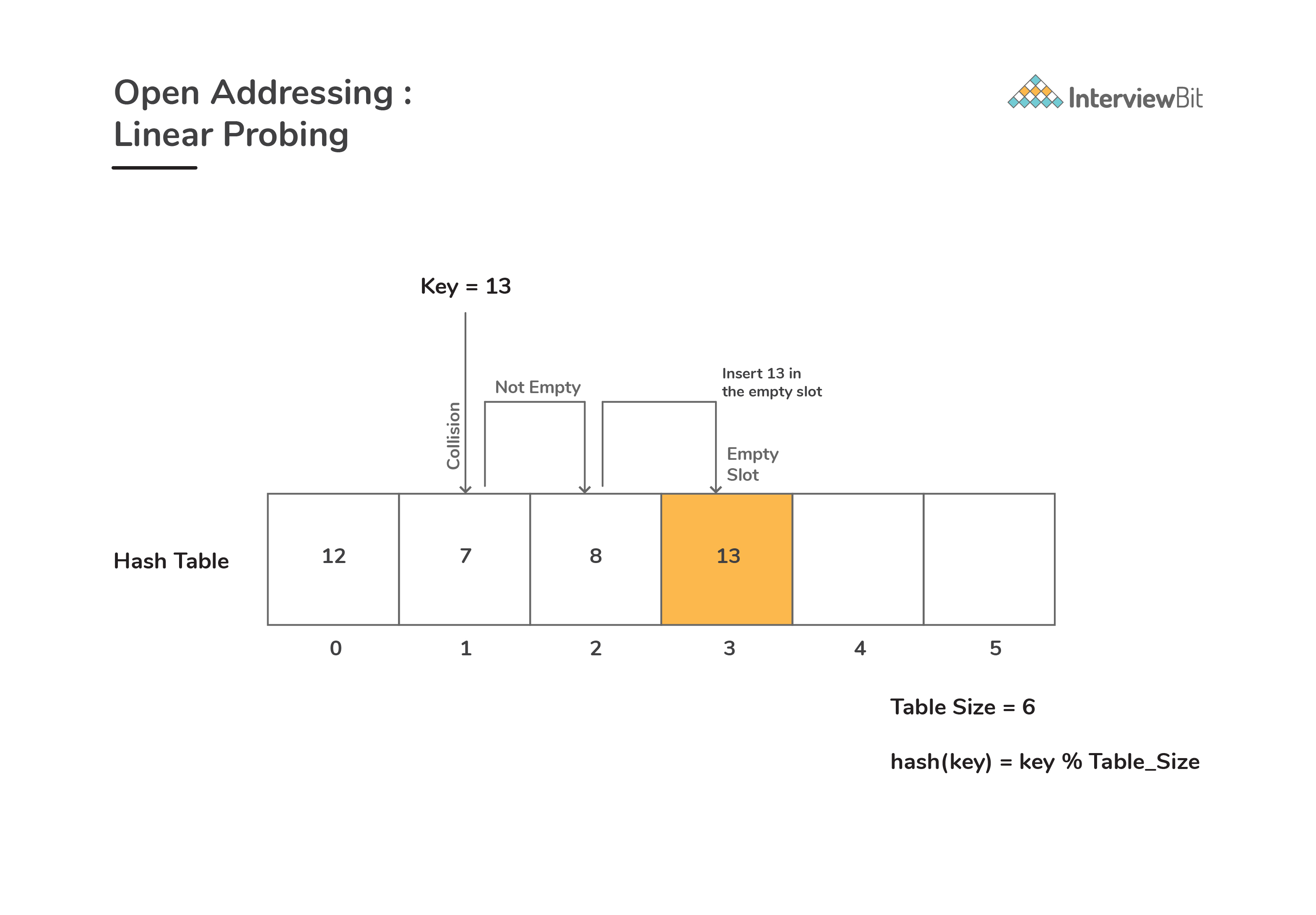• Disadvantage: There would be cases where many consecutive elements form groups and the time complexity to find the available slot or to search an element would increase greatly thereby reducing the efficiency. This phenomenon is called as clustering.

• In this approach, we look for i2-th slot in i-th iteration.
• Consider `hash(key)` be the slot index required to place key computed using hash function and `table_size` is the size of hash table available, then:
`````` If slot (hash(key) % table_size) is not free, then we check for availability of ((hash(key) + 1*1) % table_size)
If ((hash(key) + 1*1) % table_size) is again full, then we check for ((hash(key) + 2*2) % table_size)
If ((hash(key) + 2*2) % table_size) is not empty, then we check for the status of ((hash(key) + 3*3) % table_size)
:
:
:
and so on until we find the next available empty slot.
``````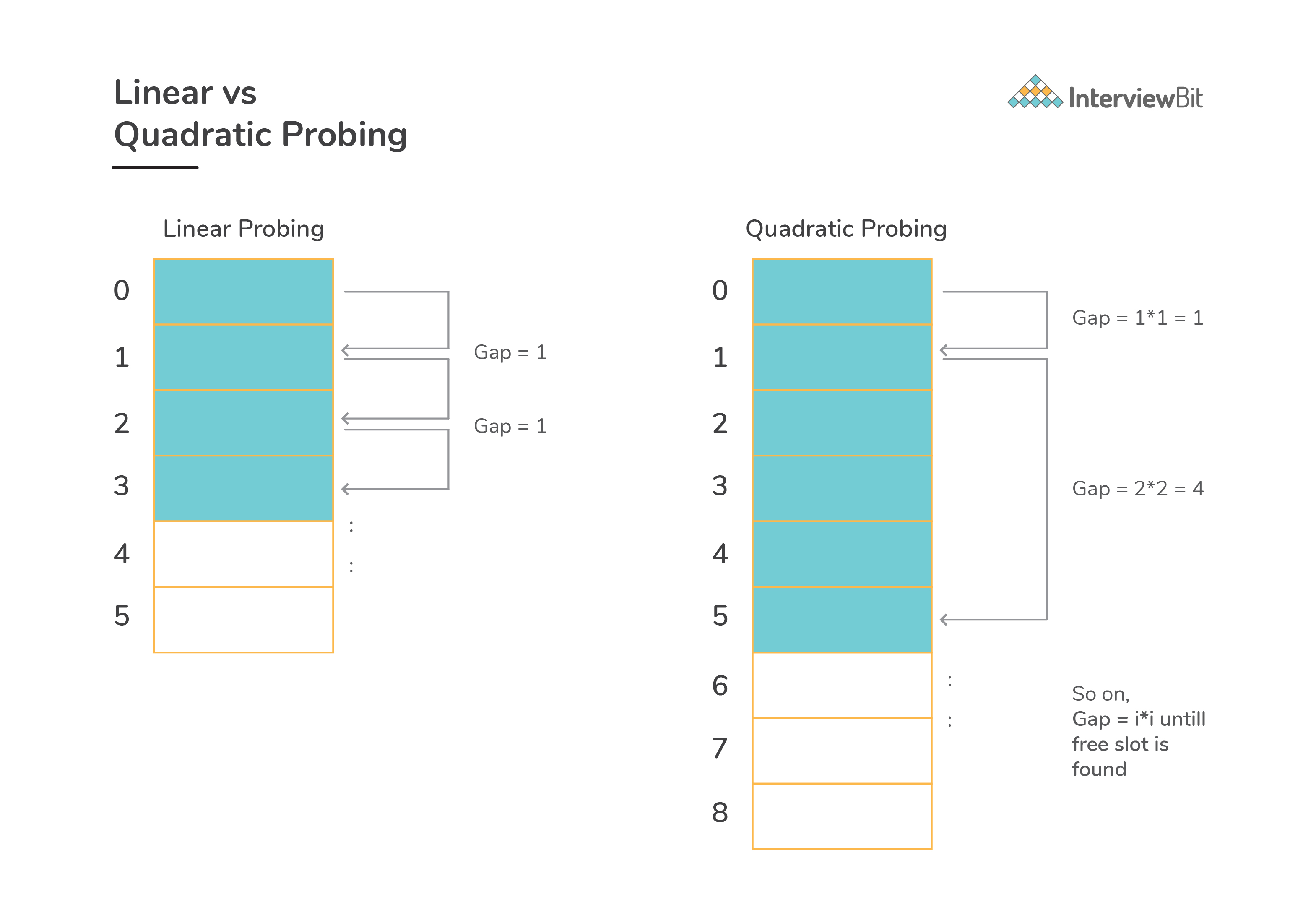• Double Hashing:

• In this method: we follow the idea of applying a second hash function on the key whenever a collision occurs.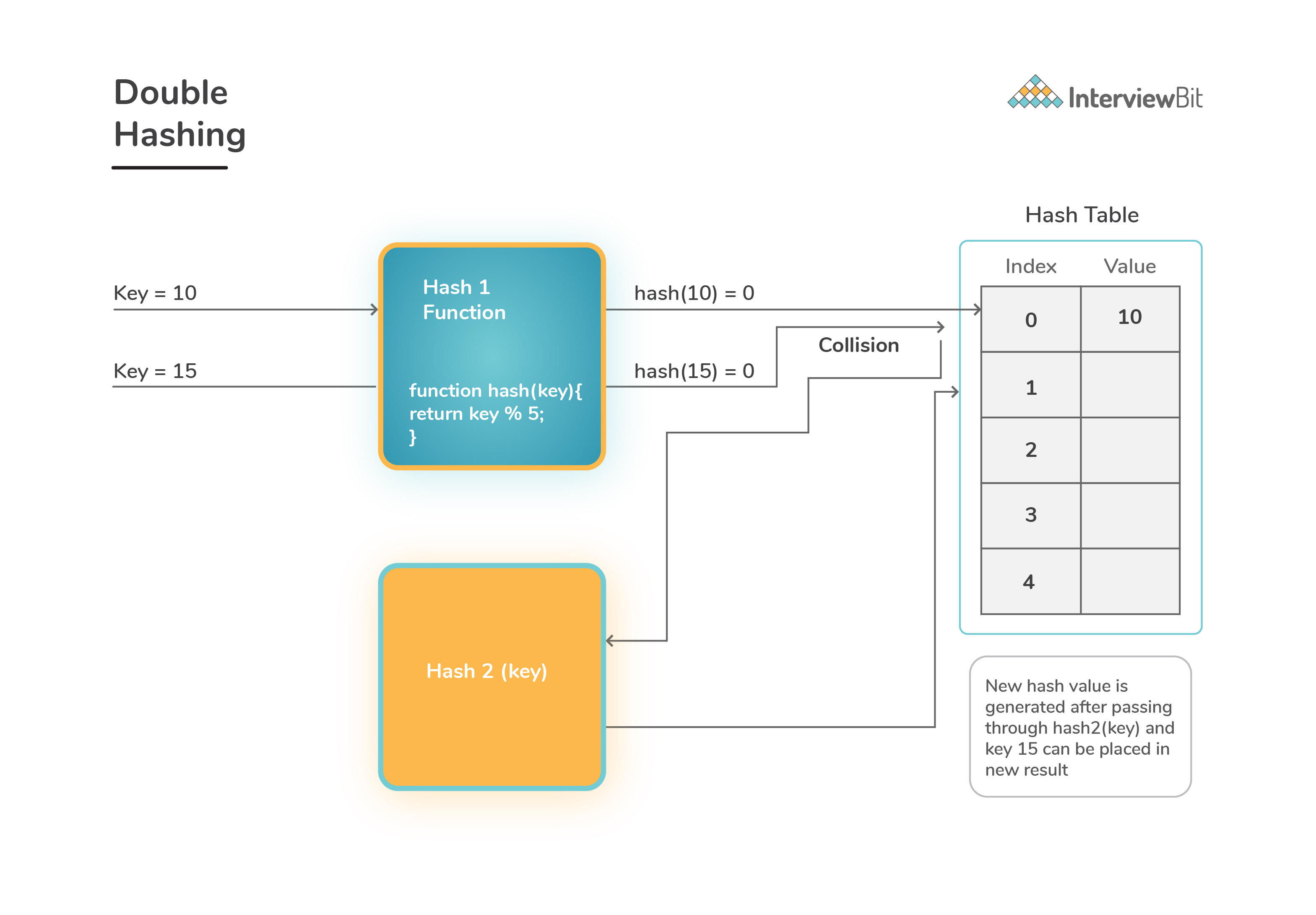• It can be done as follows:
``````(hash1(key) + c * hash2(key)) % Table_Size , where c keeps incremented by 1 upon every collision to find the next free slot.

``````
• Here `hash1()` is the first hash function and `hash2()` is the second hash function applied in case of collision and `Table_Size` is the size of hash table.
• First hash function can be defined as `hash1(key) = key % Table_Size` . Second hash function is popularly like `hash2(key) = prime_no – (key % PRIME)` where `prime_no` is a prime number smaller than `Table_Size`.
• The performance of hashing technique can be evaluated under the assumption that each key is equally likely and uniformly hashed to any slot of the hash table.
• Consider `table_size` as total number of slots in the hash table, `n` as number of keys to be inserted into the table, then:
`````` * Load factor, α = n/table_size ( α < 1 )
* Expected time taken to search/insert/delete operation < (1/(1 - α))
* Hence, search/insert/delete operations take at max (1/(1 - α)) time``````

## Serious about Learning Programming ?

Learn this and a lot more with Scaler Academy's industry vetted curriculum which covers Data Structures & Algorithms in depth.

## Hashing Problems

Hash search
Problem Score Companies Time Status
Colorful Number 150 44:55
Largest Continuous Sequence Zero Sum 200
68:44
Longest Subarray Length 200 53:50
First Repeating element 200 21:11
2 Sum 300 49:18
4 Sum 325 71:57
Valid Sudoku 325 50:22
Diffk II 375
29:05
Key formation
Problem Score Companies Time Status
Pairs With Given Xor 200 26:18
Anagrams 350 46:36
Equal 350
71:01
Copy List 450 55:56
Maths and hashing
Problem Score Companies Time Status
Check Palindrome! 200 17:48
Fraction 450 81:29
Points on the Straight Line 450 78:01
Incremental hash
Problem Score Companies Time Status
An Increment Problem 200 48:29
Subarray with given XOR 200 53:10
Two out of Three 200 32:56
Substring Concatenation 1000
71:11
Hashing two pointer
Problem Score Companies Time Status
Subarray with B odd numbers 200 49:52
Window String 350 81:37
Longest Substring Without Repeat 350 51:12Free Mock Assessment
Help us know you better for the best experience
Current Employer *
Enter company name